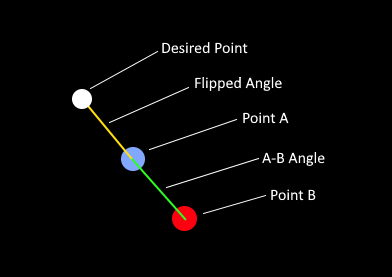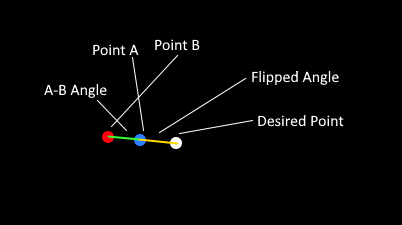Hopefully I can explain this succinctly. I have two points Point A (Blue) and Point B (Red.) I'm trying to figure out how I would go about finding the "inverse" coordinates, which would result in Desired Point (White.) If the blue point was elsewhere, then the white point would also be elsewhere, but always at the same distance, and at the same angle.

I'm sure I'm just not fluent enough in Vector maths, or maths, to figure it out. I have tried get the angle from Point A to Point B. Then I thought I could rotate that angle 180 degrees, and use the difference between Point A and B as the distance to the new point, but it don't seem to be as simple as that.

I hope I'm making sense....and apologies for the cheap diagrams.

Godot version 3.3
in Engine

``````func get_negative_vector(origin_vector, destination_vector):
I'm considering `origin_vector` to be your Point A and `destination_vector` to be your Point B. Basically, I get the line segment between the two points, rotate it 180 degrees and add it to Point A.